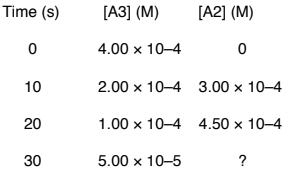# Problem: A concentration-time study of the gas phase reaction 2 A3 → 3 A2 produced the data in the table below.What is the average rate of decomposition of A3 in the time interval 20-30 seconds? Enter your answer in exponential format (example 1.23E-4) with two decimal places and no units.a. -6.2E-5b. -5.0E-6c. -4.3E-6d. +4.3E-6e. +3.2E-5

###### FREE Expert Solution

Determine the average rate of decomposition for A3 over a 20-30s time interval.

The rate of a chemical reaction is the speed at which the reactants are converted into products.

It is expressed as the change in concentrations of reactants or products over time.

The given reaction is:

2 A3 → 3 A2###### Problem Details

A concentration-time study of the gas phase reaction 2 A3 → 3 A2 produced the data in the table below.What is the average rate of decomposition of A3 in the time interval 20-30 seconds? Enter your answer in exponential format (example 1.23E-4) with two decimal places and no units.

a. -6.2E-5

b. -5.0E-6

c. -4.3E-6

d. +4.3E-6

e. +3.2E-5